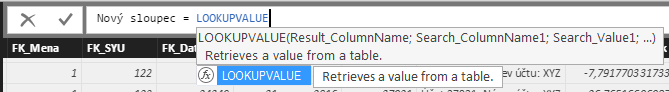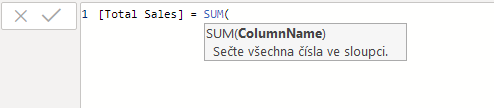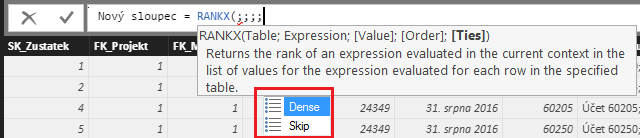• 11. 2. 2020
• Ing. Jan Zedníček - Data & Finance
• 0

DAX language haves its standards as does any other language. This article sums up and describes how look the parameters of formulas. In other words, this article is about function arguments overview.  It is good to know parameter categories appearing in this language to effectively use parameters in DAX.

## What is Function Parameter in DAX?

Demonstration – in the screenshot is function LOOKUPVALUE. It is equivalent of Excel function VLOOKUP. The function works similarly. It returns column value in table A based on match of other column in table A with value in table B.Parameters in this case are:

• Result_ColumnName – name of the column containing the value wanted to be returned using function
• Search_ColumnName – name of the column in which the value is located and based on which we search for match in column Result_ColumnName
• Search_Value – value based on which we search for match

## Parameters in DAX – Names and Descriptions

1) Expression – call sign for an expression returning scalar value. Each expression is evaluated for each row of the table. Lower is the example of sum function. It calculates total sum of all sales amounts in a table.

`[Total Sales] =``SUM ([Sales_Amount])`

But the expression can be only:`[Total Sales] =``[Sales_Amount]*2`

2) Table – expression returning other table as a result. In the example, it can be seen how to generate list of dates between 2 two bordering dates. All done using function CALENDAR.

`[Calendar] := CALENDAR(DateStart; DateEnd)`3) TableName – name of an existing table. In this case is the name of the table argument of function SUMX. We perform sum in table “Table_With_Sales” of expression Amount after discount.

`[Amount after discount] :=``SUMX ([Table_With_Sales];``[Sales_Amount]* ( 1 - [Discount %] ))`4) Column name – similar to 3) TableName – it is a name of column in existing table. It is being used as function argument – for example already mentioned LOOKUP5) Name – Text constant expressing name of new object (column in a table)6) Order – In functions, it is possible to use parameter which for any reason organizes our data in descending (DESC) or ascending (ASC) order. Example of this can be function TOPN. It returns TOP X values from any table organized by some value. We need to tell it by what it should guide itself (ASC/DESC) so it will be possible for it to return result of TOP X values.

`= TOPN (15,Employees,Employee[LastName],ASC)`7) Ties – Parameters characteristic for many advanced functions. According Excel equivalent might be last logical function parameter VLOOKUP (approximate/exact value)Rate this post
Tags:### Ing. Jan Zedníček - Data & Finance

My name is Jan Zedníček and I work as a freelancer. I have been working as a freelancer for many companies for more than 10 years.
I used to work as a financial controller, analyst and manager at many different companies in field of banking and manufacturing. When I am not at work, I like playing volleyball, chess, doing a workout in the gym.